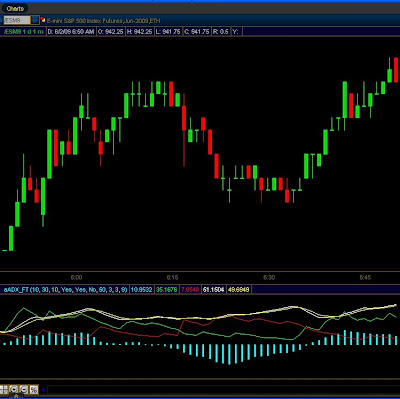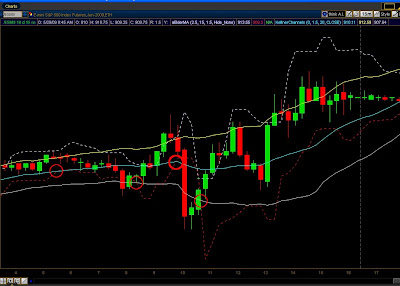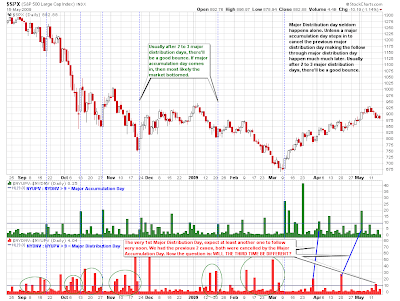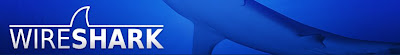## Tuesday, June 2, 2009

### Code example for referencing TOS studies for a readers emailA reader wrote in and asked a question. I'm not sure if I understood exactly what my reader wanted to do but here is one example of leveraging TOS studies.

Reference SimpleMovingAvg(length=21). That would return the SMA value for that bar. If the SMA(21) was less than the close of that bar it would print a point at the specified value.

insideBar.setHiding( if(close > reference SimpleMovingAvg(length=21),1,0));

So if(close > reference SimpleMovingAvg(length=21),1,0)) says that if close > SMA(21) then return true, else return false. Return value true activates the Hide function, return value 0 does not activate it.

(Thanks for the email. I hope you don't mind me sharing a portion of it so all can learn. Best regards, Freethinkscript).

input offset = 1 ;
plot InsideBar = if close < close then high + offset else double.NaN;

insideBar.SetPaintingStrategy(paintingStrategy.POINTS);
InsideBar.SetDefaultColor(color.LIGHT_GREEN);
insideBar.SetLineWeight(5);
insideBar.setHiding( if(close > reference SimpleMovingAvg(length=21),1,0));Developed by Dr. Martin Zweig, the Breadth Thrust Indicator measures market momentum. The Breadth Thrust is calculated by dividing a 10-day exponential moving average of the number of advancing issues, by the number of advancing plus declining issues.

A "Breadth Thrust" occurs when, during a 10-day period, the Breadth Thrust indicator rises from below 40% to above 61.5%. A "Thrust" indicates that the stock market has rapidly changed from an oversold condition to one of strength, but has not yet become overbought. According to Dr. Zweig, there have only been fourteen Breadth Thrusts since 1945. The average gain following these fourteen Thrusts was 24.6% in an average time-frame of eleven months. Dr. Zweig also points out that most bull markets begin with a Breadth Thrust.

A weekly chart is best for this indicator.declare lower;

plot zw = ExpAverage(data = close("\$advn"), length = 10 ) /( ExpAverage(data=close("\$advn"), length=10) + ExpAverage(data=close("\$decn"), length = 10));
plot forty = 0.40;
forty.SetDefaultColor(Color.RED);
plot sixfifteen = 0.615;
sixfifteen.SetDefaultColor(Color.Yellow);

### ADX, DMI, MACD tri-indicator for one low price - FREEMany times a combination of indicators can shead more light on price movement. Try this combination and see how you like it. Change the input value invertNegMACD = Yes to see a more visual picture of MACD.

You can see ADX is colored White, and also ADX Average is colored Yellow. DMI(positive) is Green, DMI(Negative) is Red, and MACD is painted as a Histogram.declare lower;
input MACDfastLen = 10;
input MACDslowLen = 30;
input MACDLen = 10;
input showADX_DMI = { "No", default "Yes"};
input showMACD = { "No", default "Yes"};
input invertNegMACD = { "Yes", default "No"};
input MACDHeight = 50;
input MACDWidth = 3;
input DMI_Len = 9;

def fastAvg = Ema2(data = close, "smoothing factor" = 2 / (1 + MACDfastLen));
def slowAvg = Ema2(data = close, "smoothing factor" = 2 / (1 + MACDslowLen));
def Value = fastAvg - slowAvg;
def nextAvg = ExpAverage(data = Value, MACDLen);
def HistoBar = value - nextAvg;
def HiScale = HighestAll(HistoBar);
def LoScale = AbsValue(LowestAll(HistoBar));
def BarScale = if HiScale > LoScale then HiScale else LoScale;

plot macd_plot = if (showMACD, if( invertNegMACD, if ( HistoBar < 0,( -1 * HistoBar * MACDHeight / BarScale ),( HistoBar * MACDHeight / BarScale )),HistoBar * MACDHeight / BarScale), double.nan);

macd_plot.AssignValueColor(if invertNegMACD then if HistoBar >= 0 then Color.Cyan else Color.Magenta else Color.Cyan);
macd_plot.SetPaintingStrategy(PaintingStrategy.Histogram);
macd_plot.SetLineWeight(MACDWidth);

def hiDiff = high - high;
def loDiff = low - low;
def plusDM = if hiDiff > loDiff and hiDiff > 0 then hiDiff else 0;
def minusDM = if loDiff > hiDiff and loDiff > 0 then loDiff else 0;
def ATR = WildersAverage(TrueRange(high, close, low), DMI_Len);
plot "DI+" =
if showADX_DMI then 100 * WildersAverage(plusDM, DMI_Len) / ATR
else double.nan;
plot "DI-" =
if showADX_DMI then 100 * WildersAverage(minusDM, DMI_Len) / ATR
else double.nan;
def DX =
if ("DI+" + "DI-" > 0) then 100 * AbsValue("DI+" - "DI-") / ("DI+" + "DI-")
else 0;

"DI+".SetDefaultColor(Color.Green);
"DI-".SetDefaultColor(Color.Red);

## Monday, June 1, 2009

### Keeping an eye on Volume Weighted MACDMACD measures the difference between two Exponential Moving Averages (EMAs). A positive MACD indicates that the 12-day EMA is trading above the 26-day EMA. On the other hand, a negative MACD indicates that the 12-day EMA is trading below the 26-day EMA. Basically, when the MACD is above the 0 line, it is indicating that the momentum is bullish. When the MACD is negative however, it is indicating that the momentum is bearish.

When the 12 days moving average crosses the 26 day moving average, you should watch the next price movements very carefully as it tends to signal a trend reversal. If the 12 days MA crosses above the 26 days MA, it’s a bullish signal. On the flip side, if the 12 days MA crosses below the 26 das MA, it’s a bearish signal.This is a good indicator to watch while markets are moving like they are now.

declare lower;

input fastLength = 12;
input slowLength = 26;
input MACDLength = 9;

def fastAvg = sum(data = (close * volume), length = fastLength)/sum(data = volume, length = fastLength);

def slowAvg = sum(data = (close * volume), length = slowLength)/sum(data = volume, length = slowLength);

plot Value = fastAvg - slowAvg;
plot Avg = ExpAverage(data = Value, length = MACDLength);

plot baseline = 0;
plot diff = value - avg;
diff.AssignValueColor (if diff >= 0 then Color.UPTICK else Color.DOWNTICK);
diff.SetPaintingStrategy(PaintingStrategy.HISTOGRAM);

diff.SetDefaultColor(GetColor(8));
Avg.SetDefaultColor(GetColor(5));
Value.SetDefaultColor(GetColor(1));
baseline.SetDefaultColor(GetColor(0));

(can also be found in default studies)

## Friday, May 29, 2009

### Bull-Bear Magic plain and simple.Apply The Ketler Channel Study to you chart with this magic. If you're a Bear watch for the red lower dashed line to cross the KC midline for a sell(in some cases you must visually project the KC midline when price action is moving quickly), exit your short when the red dashed line crosses the KC lower band.

If you're a Bully do the opposite with the upper dashed line. Enjoy.input tolerance = 1.5;
input Length = 15;
input coeff = 2.5;
input hideLines = {Hide_Hi, Hide_Lo, Hide_Both, default Hide_None};

def h_hi;
def h_lo;
switch(hideLines){
case Hide_Hi:
h_hi = 1; h_lo = 0;
case Hide_Lo:
h_hi = 0; h_lo = 1;
case Hide_Both:
h_hi = 1; h_lo = 1;
default:
h_hi = 0; h_lo = 0;
}
def hi_Avg = sum(if(high > high,high - high,0), Length) / sum(if(high > high,1,0), Length);
def hi_line = high + (hi_Avg * coeff);
def hi_max = Min(Min(hi_line, hi_line), hi_line);
plot hi_plot = hi_max;
hi_plot.setStyle(Curve.Short_Dash);
hi_plot.setHiding(h_hi);

def lo_Avg = sum(if(low < low,low - low,0), Length) / sum(if(low < low,1,0), Length);
def lo_line = low - (lo_Avg * coeff);
def lo_max = Max(Max(lo_line, lo_line), lo_line);
plot lo_plot = lo_max;
lo_plot.setStyle(Curve.Short_Dash);
lo_plot.setHiding(h_lo);

plot magic = if( (hi_max-lo_max) <= tolerance, hi_max,double.nan);
magic.setPaintingStrategy(PaintingStrategy.POINTS);

## Thursday, May 28, 2009

### Timing is everything, end false signals.A reader, Bryan wrote in last week with a common complaint about MA's.

"I want to find the best time to enter and exit an order. EMA's are great but so many times a fast line will cross a slow line but then turn right around and un-cross. I want a way to keep from entering and exiting orders to often. It seems like
whenever the fast EMA crosses the slow EMA AND a certain amount of time passes the combination of those 3 events signals a longer term trend. When I say "long" I
mean like half a day for day trading"

Here's a study for you. The indicator stays 'red' after the Moving Averages cross. When it turns green you are in the safe zone. This is great for true mechanical systems to make sure you don't enter and exit on false signals.input price = close;
input Shorter_Time = 10;
input Longer_Time = 21;

Def difference = average(data = price, length = Longer_Time) - average(data = price, length = Shorter_Time);

plot timer=price;
timer.AssignValueColor(if difference <0 then
Color.UPTICK
else
if difference >= 0 then
Color.DOWNTICK
else
GetColor(1)
);

## Wednesday, May 27, 2009You know I like Free-dom. Here is a great Webinar freebee at EminiAddict. Dave will teach all about the Ambush trading method.

Between the hours of 9:20am - 4:15pm on 05.27.09

!https://www.gotowebinar.com/register/296630163

Enjoy

## Tuesday, May 26, 2009

### Distribution, it's come a long way.A theory about Major Distribution Day; called a 90% down day is that a Major Distribution Day never comes along, so once the first one happens, there’ll be at least another one, unless a Major Accumulation Day kicks in, then the Major Distribution Day has to be recounted.

A theory about magic number three is, the 3rd time usually is different.

Now look at the chart, 2 previous Major Distribution Day, both cancelled by a Major Accumulation Day thereafter. Now this is the 3rd time we have a Major Distribution Day, so the question is: Will the 3rd time be different?Here is a Thinkscript to identify MAD's and MDD's. Both Accumulation and Distribution days. They don't happen all the time. Running this every night will make them obvious.

declare lower;

input max_distday = 9;
input accumulation_or_distribution = {"accum", default "dist"};

def uVolume = close("\$UVOL");
def dVolume = close("\$DVOL");

plot baseline = 0;
plot distribution_day = max_distday;

plot volume;

switch(accumulation_or_distribution){
case accum:
volume = uVolume / dVolume;
default:
volume = dVolume / uVolume;
}

volume.SetPaintingStrategy(PaintingStrategy.HISTOGRAM);
volume.DefineColor("Positive", Color.UPTICK);
volume.DefineColor("Negative", Color.DOWNTICK);
volume.AssignValueColor(if volume >= max_distday then volume.color("Positive") else volume.color("Negative"));### I'm a Navy Pilot. Here are my videos to prove it.

Here is a little video I took while flying my F-14 and protecting the USA. Enjoy.

Here I am flying, thinking about my wife and my 7 kids. Some clips with my dogs.

For the uninitiated, this is just a little joke. Some guy on the Internets "claims" to be a pilot. LoL, and some suckers believe him.

Look at the Red/Green dots on the candle chart.This is a nice Buy/Sell indicator that will give you good signals.

Green on Bottom = Buy Signal.
Green on Top = Keep your long until you get a Red on top signal.

Red on Top = Sell.
Red on Bottom = Stay with your short until you get a green on bottom.

input signalOffsetFactor = 0.20;

def signalOffset = AvgTrueRange(high,close,low,9)*signalOffsetFactor;plot Data = hlc3;

def triggerSell = if(if(close[-1] < high, 1, 0) and (hlc3[-2] < close[-1] or hlc3[-3] < close[-1]), 1, 0);

def triggerBuy = if(if(close[-1] > low, 1, 0) and (hlc3[-2] > close[-1] or hlc3[-3] > close[-1]), 1, 0);

def thirdBarClosed = if(IsNaN(hlc3[-3]), 0, 1);

SBS.SetStyle(curve.FIRM);
SBS.SetPaintingStrategy(paintingStrategy.LINE_VS_POINTS);
SBS.SetLineWeight(2);

SBS.AssignValueColor(if triggerSell then
if thirdbarclosed then
#UpPos
CreateColor(255, 0, 0) else
#UpNeg
CreateColor(255, 0, 0)
#DnPos
CreateColor(0, 255, 0) else
#DnNeg
CreateColor(0, 255, 0));

### CrazyFibs, Draw Fibanocci levels on anything

Here's a great new software tool. Wanna draw fibs on someones chart, or during a live webinar? Check out CrazyFibs## Saturday, May 23, 2009

### Vacation weekend puzzle

I'll leave you with a puzzle to print out for the kids. Draw your way out of the maze.Created by Jody Hall in 2001. Have a relaxing weekend.

## Friday, May 22, 2009

### ThinkOrSwim TRIX indicator as a histogram, a request from L.G.One reader asked in the comment section how to convert a standard TOS study from Line to Histogram.

Copy paste the TOS code into your own custom study and add a the function ".SetPaintingStrategy(PaintingStrategy.HISTOGRAM);" (like so)

On this study I like the Line over Histogram look, but please change it to suit your taste.

declare lower;

input length = 9;
input colorNormLength = 14;
input price = close;
input signalLength = 3;

def tr = ExpAverage(ExpAverage(ExpAverage(Log(price), length), length), length);

plot TRIX = (tr - tr) * 10000;
plot Signal = ExpAverage(TRIX, signalLength);
plot ZeroLine = 0;

def normVal = FastKCustom(AbsValue(TRIX), colorNormLength);

TRIX.SetDefaultColor(GetColor(8));
TRIX.AssignValueColor(CreateColor(255, (240 - (100 - (if TRIX > 0 then normVal else (-normVal))) * 175 / 200), 0));
ZeroLine.SetDefaultColor(GetColor(5));
Signal.setDefaultColor(GetColor(3));
TRIX.SetPaintingStrategy(PaintingStrategy.HISTOGRAM);

### A request from FreeThinkScript to you

Good morning readers. Thanks for the visit today. This morning I have a favor to ask.

Additional functionality in the thinkscript language will lead to better scripts that provide cutting edge analytical tools.

This change to the ThinkScript language is long overdue. When I "reference" a symbol from within a thinkscript I need to be able to specify the specific charting timeframe. So if I want "\$TICK" data while I'm in a 233 chart I can get "\$TICK" in a 1 minute format.

There are multiple ways to do this. One way might be to create a setter function the opposite of getAggregationPeriod().

setAggregationPeriod(_whatever_timeframe)Let's band together in a constructive fashion and ask TOS for some additional functions in the thinkscript language.

Click here for ThinkOrSwim Live chat with Tech Support. Copy/Paste this this URL and paste it into your chat session as a reference. http://freethinkscript.blogspot.com/2009/05/request-from-freethinkscript-to-you.html

Or send an email to 'tpreston@thinkorswim' and request this additional feature.

FreeThinkScript

## Thursday, May 21, 2009

### Double your Stochastics for double the fun.You can change the smoothing types: 1 = simple, 2 = exponential. Then watch when the indicators cross above 80, or below 20 for buy/sell signals.

declare lower;
declare all_for_one;

input smoothingType = 1;

def priceH = high;
def priceL = low;
def priceC = close;

input K1_Period = 5;
input K1_Slowing = 3;
input K2_Period = 8;
input K2_Slowing = 5;

def FastK_1 = (priceC - Lowest (priceL, K1_Period)) / (Highest(priceH, K1_Period) - Lowest (priceL, K1_Period)) * 100;
def FastK_2 = (priceC - Lowest(priceL, K2_Period)) / (Highest(priceH, K2_Period) - Lowest(priceL, K2_Period)) * 100;

plot Line20 = 20;
Line20.SetDefaultColor(Color.Red);
plot Line50 = 50;
Line50.SetDefaultColor(Color.White);
plot Line80 = 80;
Line80.SetDefaultColor(Color.Red);

plot FullK_2;
plot FullK_1;

if smoothingType == 1 then {
FullK_1 = Average(FastK_1, K1_Slowing);
FullK_2 = Average(FastK_2, K2_Slowing); }
else {
FullK_1 = ExpAverage(FastK_1, K1_Slowing);
FullK_2 = ExpAverage(FastK_2, K2_Slowing); }

FullK_1.SetDefaultColor(Color.Yellow);
FullK_1.SetLineWeight(1);
FullK_2.SetDefaultColor(Color.Green);
FullK_2.SetLineWeight(1);

### Technical questions about \$PREM and/or \$EPREM for ThinkOrSwimA few readers have emailed and asked if I have a script for \$PREM and/or \$EPREM for ThinkOrSwim. The answer is no. TOS does not make data for the Big S&P futures contract available and that is what you need to calculate \$PREM. If you want this kind of good data you will have to find it elsewhere.

Rules to calculate your own Premium: Subtract the Cash from the S&P front month contract (DO NOT use the minis - always use the S&P 500 futures). That will give you the "spread" between the 2.

F=S[1+(i-d)t/360]

F = Break Even Futures Price
S = Spot index price
i = interest rate (expressed as money market yeild)
d = dividend rate (expressed as money market yeild)
t = number of days from todays spot value date to the value date of the futures contract

## Wednesday, May 20, 2009

### Turtles, Snails and Tropical Fish with Thinkscript

This one is for Steve A.Well maybe not the snails and tropical fish. Tonight we'll take a look at the Turtle Trading System.

My very good friend Cho Sing Kum has a very detailed article about the World renouned Turtle Trading System. Please take a minute to review his article

Cho does use Tradestation but he is a nice guy so don't get too mad at him. Let's cut his logic over to ThinkOrSwim! Here are a couple of variations on the Donchian Channels. I run them both at once. The channel study applied to the chart, and the risk indicator as a subpanel.Create 2 seperate studies and apply them both to the same chart. The 1 minute is most reliable.
Channels

declare upper;
input length = 20;
plot topBand = Highest(high, length);
plot bottomBand = Lowest(low, length);
plot centerBand = (topBand + bottomBand) / 2;

topBand.SetDefaultColor(Color.BLUE);
bottomBand.SetDefaultColor(Color.BLUE);
centerBand.SetDefaultColor(Color.BLUE);

Risk tolerance
Let's say you only wanted to risk \$1000 on a Emini trade.
Enter cash=\$1000 and this lower study will show you breakout channels based on your risk tolerance. Make sure that the 'Length' variable is the same in both studies.
Awesome free thinkscripts for thinkorswim!

declare lower;
input length=20;
input cash =1000;
input valueLine = 500;
plot channel = (Highest(High,length)-Lowest(low,length))*cash;

channel.setdefaultColor(Color.DOWNTICK);
plot data1 = cash;
data1.setDefaultColor(Color.YELLOW);
plot data2 = valueLine;
data2.setDefaultColor(Color.BLUE);

### Free Thinkscript code for breakpointtrades.com Mechanical Systems

Here is a good source of quality information on charting, and mechanical systems.

Here is a sample of their daily update:

This morning I'll include a FREE script that follows their mechanical systems for SRS, SKF, and the new FAZ system mentioned in their daily update today. I'll add Chris' clouds to it as I know you guys like the pretty clouds.Here is what we have:And finally the code:

declare upper;
input price = close;
input displace = 0;

input EMALength1 = 9;
input EMALength2 = 39;

plot upper = ExpAverage(data = price[-displace], length = EMALength1);
upper.SetDefaultColor(Color.RED);
plot lower = ExpAverage(data = price[-displace], length = EMALength2);
lower.SetDefaultColor(Color.BLUE);

Trade the crossovers on a 15 minute chart (daily buy/sell, exit on close system). In the link above at about the 9:05 minute mark in the audio you can hear all about it.
SRS - 9/39 EMA
SKF - 29/86 EMA (so change EMALength1 to 29, EMALength2 to 86 on a 5 min chart)

A little music to start you trading day.

## Tuesday, May 19, 2009

### Larry Williams%R indicator marries SMA indicator. Honeymoon photo included.The Williams%R indicator is famous for the -20 and -80 % levels to indicate overbought and oversold conditions. Watch for the 2 lines to cross on the -20 and -80 centerlines for your potential signals.

Enjoy the Free code.

declare lower;

input Length = 14;
input OverBought = -20;
input OverSold = -80;
input MovAvgLength = 9;

def highest = Highest(high, Length);
def lowest = Lowest(low, Length);
def data = if highest == lowest then -100 else (highest - close) / (highest - lowest) * (-100);

plot PercentR_SMA = if( data > 0, 0, data);
PercentR_SMA.SetDefaultColor(Color.BLUE);
plot Over_Sold = overSold;
Over_Sold.SetDefaultColor(GetColor(8));
plot Over_Bought = overBought;
Over_Bought.SetDefaultColor(GetColor(8));
plot SMA = Average(data =PercentR_SMA, length = MovAvgLength);
SMA.SetDefaultColor(GetColor(5));

### Easter egg hunting with ThinkOrSwim?

I've received a few emails asking if I know more about thinkscript than what is provided in the documentation. The answer is yes. In the coming weeks I will post some interesting facts and conclusions about the application that we all run on our computers.

One such question comes up in the native TOS PivotPoint study. TOS's hidden PivotPoint code. "why does TOS hide their PivotPoint code". If it's a simple (Hi+Lo+Close)/3 calculation as John Person describes why hide the code? It's all here: http://www.nationalfutures.com/pivotcalculator.htm

http://mediaserver.thinkorswim.com/notices/Release_020609.htmlCan't wait? You want to find out if you can find any Easter Eggs hidden? OK. Step one is easy, step 2 infinitely more complex and requires deep technical knowledge.

Step 1:
If you don't already have Wireshark. Download and install this utility. It allows you to examine the data that is sent to and from the servers that you communicate with on the Internet.
In this communication stream you can find a lot of useful information about what data is sent and received, vs. what data you see on the application.

http://www.wireshark.orgStep 2:
The second step is to run your financial software package on ReactOS. ReactOS is the OpenSource Windows Operating System. The advantage of doing this is that you can also be running a kernel level debugger hooked directly into the software that you are running and set breakpoints to enhance discovery.Look for my upcoming articles about various aspects of the TOS software platform and the traders edge.